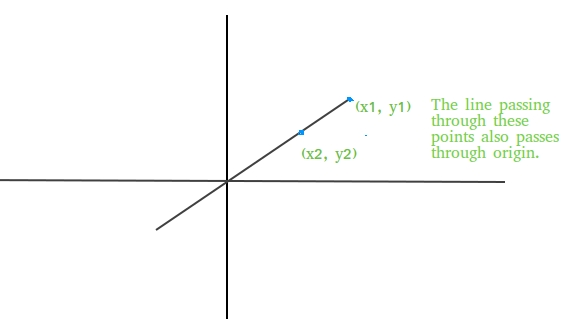# Check if a line passes through the origin

• Difficulty Level : Easy
• Last Updated : 16 Jun, 2022

Given two coordinates of a line as (x1, y1) and (x2, y2), find if the line passing through these points also passes through origin or not.Examples:

```Input : (x1, y1) = (10, 0)
(x2, y2) = (20, 0)
Output : Yes
The line passing through these points
clearly passes through the origin as
the line is x axis.

Input : (x1, y1) = (1, 28)
(x2, y2) = (2, 56)
Output : Yes```

Approach: Equation of a line passing through two points (x1, y1) and (x2, y2) is given by
y-y1 = ((y2-y1) / (x2-x1))(x-x1) + c
If line is also passing through origin, then c=0, so equation of line becomes
y-y1 = ((y2-y1) / (x2-x1))(x-x1)
Keeping x=0, y=0 in the above equation we get,
x1(y2-y1) = y1(x2-x1)
So above equation must be satisfied if any line passing through two coordinates (x1, y1) and (x2, y2) also passes through origin (0, 0).

## C++

 `/* C++ program to find if line passing through``   ``two coordinates also passes through origin``   ``or not */``#include ``using` `namespace` `std;` `bool` `checkOrigin(``int` `x1, ``int` `y1, ``int` `x2, ``int` `y2)``{``   ``return` `(x1 * (y2 - y1) == y1 * (x2 - x1));``}` `// Driver code``int` `main()``{``    ``if` `(checkOrigin(1, 28, 2, 56) == ``true``)``      ``cout << ``"Yes"``;``    ``else``      ``cout << ``"No"``;``    ``return` `0;``}`

## Java

 `// Java program to find if line passing through``// two coordinates also passes through origin``// or not``import` `java.io.*;` `class` `GFG {``    ` `    ``static` `boolean` `checkOrigin(``int` `x1, ``int` `y1,``                                       ``int` `x2, ``int` `y2)``    ``{``        ``return` `(x1 * (y2 - y1) == y1 * (x2 - x1));``    ``}` `    ``// Driver code``    ``public` `static` `void` `main (String[] args)``    ``{``        ``if` `(checkOrigin(``1``, ``28``, ``2``, ``56``) == ``true``)``            ``System.out.println(``"Yes"``);``        ``else``            ``System.out.println(``"No"``);``    ``}``}` `// This code is contributed by Ajit.`

## Python3

 `# Python program to find if line``# passing through two coordinates``# also passes through origin or not` `def` `checkOrigin(x1, y1, x2, y2):``    ``return` `(x1 ``*` `(y2 ``-` `y1) ``=``=` `y1 ``*` `(x2 ``-` `x1))``    ` `# Driver code``if` `(checkOrigin(``1``, ``28``, ``2``, ``56``) ``=``=` `True``):``    ``print``(``"Yes"``)``else``:``    ``print``(``"No"``)``    ` `# This code is contributed``# by Anant Agarwal.`

## C#

 `// C# program to find if line passing through``// two coordinates also passes through origin``// or not``using` `System;` `class` `GFG {` `    ``static` `bool` `checkOrigin(``int` `x1, ``int` `y1,``                            ``int` `x2, ``int` `y2)``    ``{``        ``return` `(x1 * (y2 - y1) == y1 * (x2 - x1));``    ``}` `    ``// Driver code``    ``public` `static` `void` `Main()``    ``{``        ``if` `(checkOrigin(1, 28, 2, 56) == ``true``)``            ``Console.WriteLine(``"Yes"``);``        ``else``            ``Console.WriteLine(``"No"``);``    ``}``}` `// This code is contributed by vt_m.`

## PHP

 ``

## Javascript

 ``

Output:

`Yes`

Time Complexity: O(1)
Auxiliary Space: O(1)